Next: Incompressible Inviscid Flow Up: Surface Tension Previous: Axisymmetric Soap-Bubbles

# Exercises

1. Show that if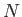equal spheres of water coalesce so as to form a single spherical drop then the surface energy is decreased by a factor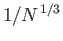. (Lamb 1928.)

2. A circular cylinder of radius, height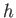, and specific gravity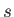floats upright in water. Show that the depth of the base below the general level of the water surface iswhere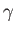is the surface tension at the air/water interface, andthe contact angle of the interface with the cylinder. (Lamb 1928.)

3. A film of water is held between two parallel plates of glass a small distance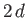apart. Prove that the apparent attraction between the plates is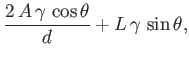whereis the surface tension at the air/water interface,the angle of contact of the interface with glass,the area of the film, andthe circumference of the film. (Lamb 1928.)

4. Show that if the surface of a sheet of water is slightly corrugated then the surface energy is increased by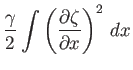per unit breadth of the corrugations. Here,is measured horizontally, perpendicular to the corrugations. Moreover,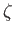denotes the elevation of the surface above the mean level. Finally,is the surface tension at an air/water interface. If the corrugations are sinusoidal, such thatshow that the average increment of the surface energy per unit area is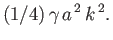(Lamb 1928.)

5. A mass of liquid, which is held together by surface tension alone, revolves about a fixed axis at a small angular velocity, so as to assume a slightly spheroidal shape of mean radius. Prove that the ellipticity of the spheroid iswhere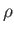is the uniform mass density, andthe surface tension. [Ifis the maximum radius, andthe minimum radius, then, and the ellipticity is defined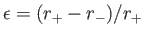.] (Lamb 1928.)

6. A liquid mass rotates, in the form of a circular ring of radiusand small cross-section, with a constant angular velocity, about an axis normal to the plane of the ring, and passing through its center. The mass is held together by surface tension alone. Show that the section of the ring must be approximately circular. Demonstrate thatwhereis the density,the surface tension, and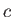the radius of the cross-section. (Lamb 1928.)

7. Two spherical soap bubbles of radiiandare made to coalesce. Show that when the temperature of the gas in the resulting bubble has returned to its initial value the radiusof the bubble satisfies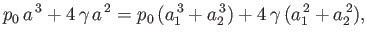where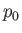is the ambient pressure, andthe surface tension of the soap/air interfaces. (Batchelor 2000.)

8. A rigid sphere of radiusrests on a flat rigid surface, and a small amount of liquid surrounds the contact point, making a concave-planar lens whose diameter is small compared to. The angle of contact of the liquid/air interface with each of the solid surfaces is zero, and the surface tension of the interface is. Show that there is an adhesive force of magnitude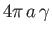acting on the sphere. (It is interesting to note that the force is independent of the volume of liquid.) (Batchelor 2000.)

9. Two small solid bodies are floating on the surface of a liquid. Show that the effect of surface tension is to make the objects approach one another if the liquid/air interface has either an acute or an obtuse angle of contact with both bodies, and to make them move away from one another if the interface has an acute angle of contact with one body, and an obtuse angle of contact with the other. (Batchelor 2000.)Next: Incompressible Inviscid Flow Up: Surface Tension Previous: Axisymmetric Soap-Bubbles
Richard Fitzpatrick 2016-03-31